Notes on BRST I: Representation Theory and Quantum Mechanics

This is the first posting of a planned series that will discuss the BRST method for handling gauge symmetries and related mathematical topics. I’ve been writing a more formal paper about this, but given the substantial amount of not-well-known background material involved, it seems like a good idea to first put together a few expository accounts of some of these topics. And what better place for this than a blog?

Many readers who are used to my usual attempts to be newsworthy and entertaining, often by scandal-mongering or stirring up trouble of one kind or another, may be very disappointed in these posts. They’re quite technical, hard to follow, and of low-to-negative entertainment value. You probably would do best to skip them and wait for more of the usual fare, which should continue to appear from time to time.

Quantum Mechanics and Representation Theory

A quantum mechanical physical system is given by the following mathematical structure:

• A Hilbert space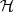, the “space of states”. A state of the physical system is determined by a vector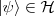, with unit norm (i.e.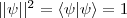).
• An algebra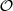that acts on. To each physical observable corresponds a self-adjoint operator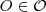. Eigenvectors inof this operator correspond to states where the observable has a well-defined value, which is the eigenvalue.
• If a physical system has a symmetry group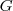, there is a unitary representationofon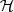. This means that for each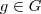we get a unitary operator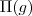satisfying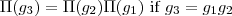i.e. the map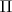from group elements to unitary operators is a homomorphism. Theact onby taking an operator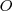to its conjugate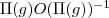.

Whenis a Lie group with Lie algebra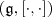, differentiatinggives a unitary representation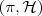of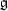on. This means that for each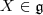we get a skew-Hermitian operator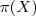on, satisfyingi.e. the maptaking Lie algebra elements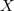(with the Lie bracket in) to skew-Hermitian operators (with commutator of operators) is a homomorphism. On,acts by the differential of the conjugation action of, this action is just that of taking the commutator with.

The Lie bracket is not associative, but to any Lie algebra, one can construct an associative algebra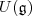called the universal enveloping algebra for. If one identifieswith left-invariant vector fields on, which are first-order differential operators on functions on, thenis the algebra of left-invariant differential operators onof all orders, with product the composition of differential operators. A Lie algebra representation is precisely a module over, i.e. a vector space with an action of.

So, the state spaceof a quantum system with symmetry groupcarries not only a unitary representation of, but also a unitary representation of, or equivalently, an action of the algebra.acts by the operator. In this way a representationgives a sub-algebra of the algebraof observables. Most of the important observables that show up in practice come from a symmetry in this way. An interesting philosophical question is whether the quantum system that governs the real world is purely determined by symmetry, i.e. such that ALL its observables come from symmetries in this manner.

Some Examples

Much of the structure of common quantum mechanical systems is governed by the fact that they carry space-time symmetries. In our 3-space, 1-time dimensional world, these include:

• Translations in space: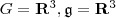, Lie Bracket is trivial.
For each basis element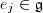one gets a momentum operator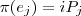• Translations in time:. Ifis a basis of,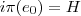, the Hamiltonian operator. The fact that this operator generates time-translations is just Schrodinger’s equation.
• Rotations in 3-space: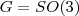, or its double cover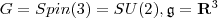, with bracket given by the vector product. For each basis elementone gets an angular momentum operator. These operators do not commute, so cannot be simultaneously diagonalized.
• Another example is the symmetry of phase transformations of the state space. Here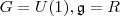, and one gets an operator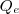that can be normalized to have integral eigenvalues.

This last example also comes in a local version, where we make independent phase transformations at different points in space-time. This is an example of a “gauge symmetry”, and the question of how it gets represented on the space of states is what will lead us into the BRST story. Next posting in the series will be about gauge symmetry, then on to BRST.

If you want some idea of where this is headed, you can take a look at slides from a colloquium talk I gave recently at the Dartmouth math department. They’re very sketchy, the postings in this series should add some detail.

This entry was posted in BRST. Bookmark the permalink.

63 Responses to Notes on BRST I: Representation Theory and Quantum Mechanics

1. Shantanu says:

Peter, this looks interesting.
Do you have any students or colleagues working with you on this?

2. Hendrik says:

Thomas,

I’m afraid I don’t follow your reasoning;- it seems you are proposing a different definition of “symmetry” but I cannot see what job your symmetry is supposed to do:

> “This suggest one definition of “symmetry”. Let G be the full group of automorphisms on the Hilbert space, and let H be the biggest subgroup such that the rep of G is induced from H. Then H is a “symmetry”, in the sense that it is the largest group that says something nontrivial about the Hilbert space.”

Is the “full group of automorphisms on the Hilbert space” all of U(H), or is it the unitaries which implements the largest set of aotomorphisms of your algebra of observables A? In short, given A how do you get G?

“…it is the largest group that says something nontrivial about the Hilbert space”. Since all Hilbert spaces of the same dimension are isomorphic, I cannot imagine what such a nontrivial property might be. Do you mean the rep of A on it?

Maybe I was a bit unclear earlier on;- in a nutshell, I think a symmetry is a transformation of the algebra of observables with a job to do. That job is to preserve the defining physical structures of the system. These strucures can be external to the algebra, e.g. a distinguished group action, or some map from space-time regions to subalgebras encoding causality/covariance etc. In this case, not all automorphisms of A can be symmetries.

Tomate,

A “conservation law” is an additive quantity;- e.g. you add up all the momenta of a set of interacting particles, and if the total set of particles is free, then the total momentum is conserved. That is why we take the generators S of the unitary groups U(t) = exp(itS) of the symmetry groups to get our conservation laws, since by the exponential law we have U(t)U(t’) = exp(i(t+t’)S). Parity is a multiplicative quantum number, not an additive one, so not a conservation law in the usual sense. Of course, given a set of (anti)unitaries implementing a discrete symmetry, commuting with time evolution, then it is easy to make conserved observables (staying constant in time);- just take any selfadjoint element in the *-algebra generated by the (anti)unitaries. However, these do not give you a conservation law in the usual sense for your original discrete symmetries.

We need a philosopher to sort out “symmetry is an unobservable degree of freedom” but let me have a stab at it. I think “to observe” is relative, and one should be clear where your observer is. Moreover you should also distinguish between applying your symmetry to to subsystems from application of it to the full system.
E.g. translation of a subsystem is an observable transformation (and physical thing to do) for an observer in the full system, but it is unobservable for an observer inside the subsystem (who by definition cannot see anything outside his system). Hence we can measure momentum of subsystems of our world, but we cannot measure the total momentum of the universe.
Thus concerning parity, we can measure it for subsystems of our world, but (if it is a symmetry for those systems), of course it is unobservable from within those systems. By the way, parity is broken by weak interactions, so perhaps that was not the best example for your point

3. tomate says:

Hendrick,

maybe this is just a philosophycal hence not scientific matter and this by definition is no place for unscientific stuff, so forgive me all. The sentence “symmetry unobservable” is uncritically stuck in my mind due to many sources none of which I’m able to mention at the moment (but could do with a little research): they might not be very authoritative (aside: can we think of a quantum experiment that tests time invariance without appealing to energy conservation? If so then I’m easily wrong). We can better say that symmetry is what leaves transition probabilities unaltered, as is in the spirit of Wigner theorem.
I just notice that symmetry is rather abstract (lagrangian invariant under such and such transformation), and conservation very practical (something calculable before experiment = something calculable after). I’m just not very comfortable with the idea that multiplicative quantum numbers are excluded from the Gotha of conserved quantities, yet sharing this very intuitive picture. You yourself put, for instance, parity and momentum conservation on the same footing in your example.
I guess it’s just a matter of convention of no conceptual importance. It would just be be more practical to say: all symmetries lead to conserved quantities. But it doesn’t seem to be worthy a referendum.
(I do know parity is an unfortunate example – but it’s the only one I remember how to calculate)

Hendrik

Symmetry is perhaps not a good word, since it has an established meaning (commutes with the Hamiltonian). But what I am saying is that it is a good idea to look at the action of ever bigger groups on the Hilbert space (or perhaps an enlarged Hilbert space), as long as this gives you new information about your system. You should stop at the largest group H such that the action is not induced from some subgroup, because for bigger groups all info is already encoded in H.

Main example: CFT in 2D. The infinite conformal symmetry G is generated by L_m = z^(m+1) d/dz. Denote by H the non-positive (m &lt= 0) subalgebra. One may argue that only H is a symmetry (note that the Hamiltonian is not known), because the positive generators diverge at infinity and thus violate boundary conditions. The Hilbert space carries a direct sum of one-dimensional reps of H, characterized by L_0 |h> = h |h>, L_-m |h> = 0.

We can extend this action to an action of all of G, at the cost of adding new states to our Hilbert space. If the action of G on the enlarged Hilbert space were induced from H, i.e. a Verma module, this exercise is pointless; essentially all info about the Verma module already lives in H. But for physically interesting systems (minimal models), the action is something different: the Verma module modulo singular vectors. This yields new info which is physically important: the discrete spectrum of critical exponents. So in this case, going from H to G is a good idea, even if it does not commute with the (unknown) Hamiltonian.

And yes, I have glitched over some details such as the central charge.

5. Aaron said : “The point is that physics deals with projective representations. The half-integral spin reps or SU(2) are perfectly good projective representations of SO(3).”

Couldn’t we take the POV that (quantum) physics deals with algebra representations instead ? The natural/”real”/important object here would be the lie algebra of SO(3) = Lie alg of SU(2). This POV would also naturally leads to quantum groups as a possible generalization.

6. Peter Woit says:

Shantanu,

The BRST project is just me.

7. Aaron Bergman says:

Couldn’t we take the POV that (quantum) physics deals with algebra representations instead ? The natural/”real”/important object here would be the lie algebra of SO(3) = Lie alg of SU(2). This POV would also naturally leads to quantum groups as a possible generalization.

Just looking at the Lie algebra doesn’t go far enough. You need to look at central extensions of the Lie algebra. It so happens that central extensions of semisimple Lie algebras aren’t particularly exciting, but there’s more to life than semisimple things. Like loop groups, say.

8. AM says:

Thanks for the post. More posts along these lines would be great !

9. Pingback: A semana nos arXivs… « Ars Physica

10. Gen Zhang says:

I realise I’m coming a bit late to this party, but:

You state, as most elementary texts do, that symmetries are implemented as a unitary representation of the group on the Hilbert space. However, it is important to realise that this is often not possible; as has been alluded to by Aaron, we really care about projective representations. Usually at this point the discussion veers off into the rough, but I’d like to bring up an elementary example: a single 2D electron in a uniform magnetic field. The system is obvious symmetric wrt. translations in the plane, but it is also easy to see (Aharonov-Bohm effect) that it is not a proper representation on the wavefunction.

I have rarely seen this issue being discussed — perhaps because the theory of projective representations is not as advanced yet. It is still surprising the number of physicists who are completely unaware of this issue though.

11. Peter Woit says:

Gen Zhang,

The point about projective representations is an important one, which I was avoiding for simplicity here. It will appear when I try and write something about affine Kac-Moody Lie algebras and their representations. Another way of dealing with this is to say that, instead of a projective representation, one ends up having a representation of an extension of the symmetry group one started with.

12. Pingback: best of 2008 (2) : big theorems

13. Blair Smith says:

No fair PW! You hang that philosophical comment (before “Some Examples”) out there like a musical note in tension without providing a resolution 😉

Maybe I need to read further on to see where you are going, but might it be possible to give that idea more teeth by say associating (via some ontological-like constraints on possible models of reality) classes of theories that alternatively do (do not) have all observables arising from symmetry groups with unitary reps on H?